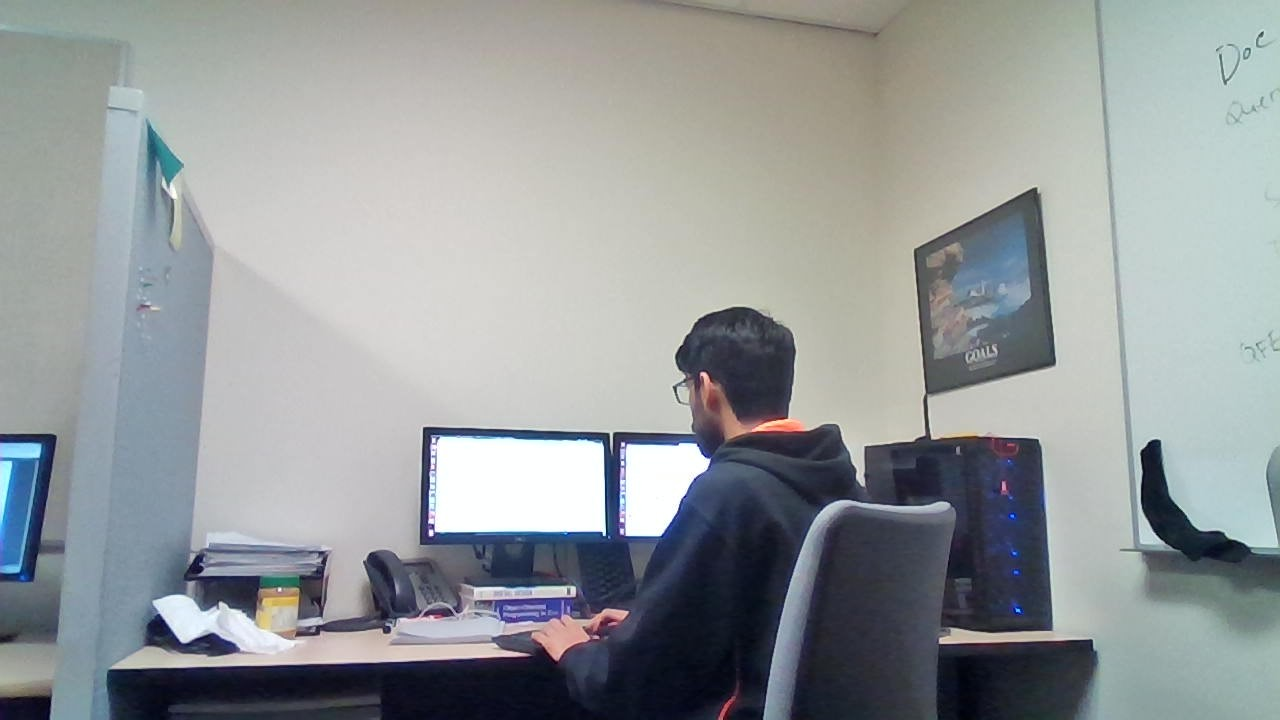# Alternate Fibonacci Numbers

Give a number N, print alternate fibonacci numbers till n-th Fibonacci.

Examples:

```Input :  N = 7
Output : 0 1 3 8

Input  : N = 15
Output : 0 1 3 8 21 55 144 377
```

## Recommended: Please try your approach on {IDE} first, before moving on to the solution.

Read method 2 in the following article : fibonacci number

Approach:Using dynamic programming approach. Keep storing the previously calculated fibonacci numbers and using the previous two to store the next fibonacci number.

## C++

 `// Alternate Fibonacci Series using Dynamic Programming ` `#include ` `using` `namespace` `std; ` ` `  `void` `alternateFib(``int` `n) ` `{ ` `    ``if` `(n < 0) ` `      ``return``; ` ` `  `    ``/* 0th and 1st number of the series are 0 and 1*/` `    ``int` `f1 = 0; ` `    ``int` `f2 = 1; ` ` `  `    ``cout << f1 << ``" "``; ` `    ``for` `(``int` `i = 2; i <= n; i++) { ` `         ``int` `f3 = f2 + f1; ` `           `  `         ``if` `(i % 2 == 0) ` `            ``cout << f3 << ``" "``; ` ` `  `         ``f1 = f2; ` `         ``f2 = f3; ` `    ``} ` `} ` ` `  `int` `main() ` `{ ` `    ``int` `N = 15; ` `    ``alternateFib(N); ` `    ``return` `0; ` `} `

## Java

 `// Alternate Fibonacci Series  ` `// using Dynamic Programming ` `import` `java.io.*; ` ` `  `class` `GFG  ` `{ ` `static` `void` `alternateFib(``int` `n) ` `{ ` `    ``if` `(n < ``0``) ` `    ``return``; ` ` `  `    ``/* 0th and 1st number of the ` `       ``series are 0 and 1*/` `    ``int` `f1 = ``0``; ` `    ``int` `f2 = ``1``; ` ` `  `    ``System.out.print(f1 + ``" "``); ` `    ``for` `(``int` `i = ``2``; i <= n; i++)  ` `    ``{ ` `        ``int` `f3 = f2 + f1; ` `         `  `        ``if` `(i % ``2` `== ``0``) ` `            ``System.out.print(f3 + ``" "``); ` ` `  `        ``f1 = f2; ` `        ``f2 = f3; ` `    ``} ` `} ` ` `  `// Driver Code ` `public` `static` `void` `main (String[] args)  ` `{ ` `    ``int` `N = ``15``; ` `    ``alternateFib(N); ` `} ` `} ` ` `  `// This code is contributed ` `// by chandan_jnu. `

## Python3

 `# Alternate Fibonacci Series ` `# using Dynamic Programming ` `def` `alternateFib(n): ` `    ``if` `(n < ``0``): ` `        ``return` `-``1``; ` ` `  `    ``# 0th and 1st number of ` `    ``# the series are 0 and 1 ` `    ``f1 ``=` `0``; ` `    ``f2 ``=` `1``; ` ` `  `    ``print``(f1, end ``=` `" "``); ` `    ``for` `i ``in` `range``(``2``, n ``+` `1``): ` `        ``f3 ``=` `f2 ``+` `f1; ` `         `  `        ``if` `(i ``%` `2` `=``=` `0``): ` `            ``print``(f3, end ``=` `" "``); ` ` `  `        ``f1 ``=` `f2; ` `        ``f2 ``=` `f3; ` ` `  `# Driver Code ` `N ``=` `15``; ` `alternateFib(N); ` ` `  `# This code is contributed by mits `

## C#

 `// Alternate Fibonacci Series  ` `// using Dynamic Programming ` `using` `System; ` ` `  `class` `GFG  ` `{ ` `static` `void` `alternateFib(``int` `n) ` `{ ` `    ``if` `(n < 0) ` `    ``return``; ` ` `  `    ``/* 0th and 1st number of  ` `    ``the series are 0 and 1*/` `    ``int` `f1 = 0; ` `    ``int` `f2 = 1; ` ` `  `    ``Console.Write(f1 + ``" "``); ` `    ``for` `(``int` `i = 2; i <= n; i++)  ` `    ``{ ` `        ``int` `f3 = f2 + f1; ` `         `  `        ``if` `(i % 2 == 0) ` `            ``Console.Write(f3 + ``" "``); ` ` `  `        ``f1 = f2; ` `        ``f2 = f3; ` `    ``} ` `} ` ` `  `// Driver Code ` `public` `static` `void` `Main ()  ` `{ ` `    ``int` `N = 15; ` `    ``alternateFib(N); ` `} ` `} ` ` `  `// This code is contributed ` `// by chandan_jnu. `

## PHP

 ` `

Output:

```0 1 3 8 21 55 144 377
```

Time Complexity : O(n)
Auxiliary Space : O(1)

Attention reader! Don’t stop learning now. Get hold of all the important DSA concepts with the DSA Self Paced Course at a student-friendly price and become industry ready.

My Personal Notes arrow_drop_upCheck out this Author's contributed articles.

If you like GeeksforGeeks and would like to contribute, you can also write an article using contribute.geeksforgeeks.org or mail your article to contribute@geeksforgeeks.org. See your article appearing on the GeeksforGeeks main page and help other Geeks.

Please Improve this article if you find anything incorrect by clicking on the "Improve Article" button below.